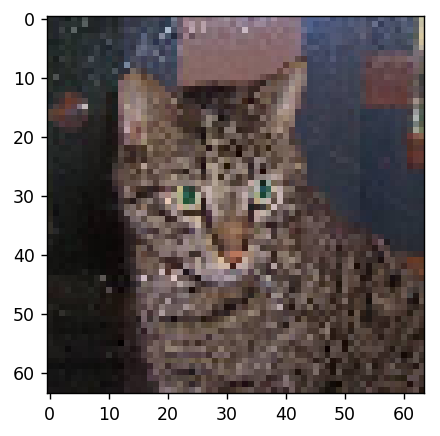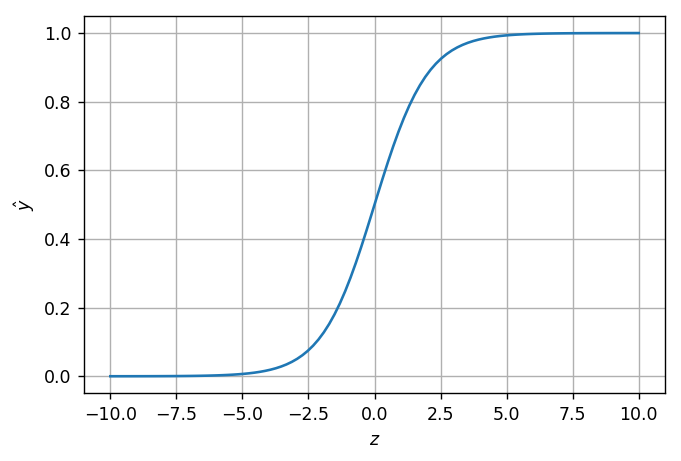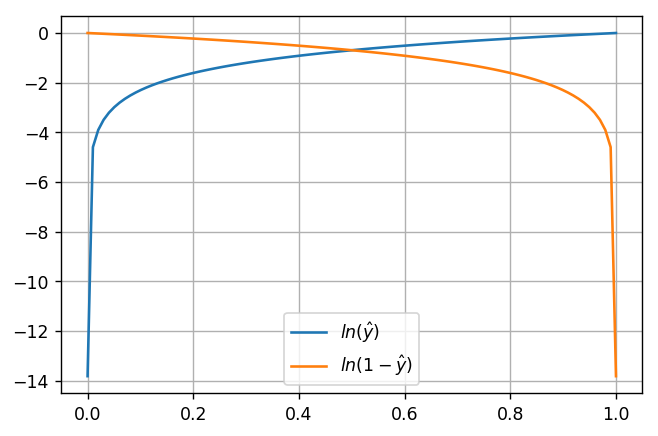# Machine Learning 101¶

KharkivPy #17
November 25th, 2017

by Roman Podoliaka, Software Engineer at DataRobot

email: [email protected]
blog: http://podoliaka.org
slides: http://podoliaka.org/talks/

## Goal¶

Take a close look at one of the simplest machine learning algorithms - logistic regression - to understand how it works internally and how it can be applied for the task of image classification.

... and, hopefully, dispel at least some hype around machine learning.

## Problem¶

Create an algorithm (also called a model) to classify whether images contain either a dog or a cat.Kaggle competition: https://www.kaggle.com/c/dogs-vs-cats

There are much better algorithms for this task, but we'll stick to logistic regression for the sake of simplicity.

## Input data¶

25000 labeled examples of JPEG images (of different sizes) of cats and dogs, e.g.: cat.0.jpg, cat.1.jpg, ..., dog.1.jpg, dog.2.jpg...## Terminology and notation¶

This task is an example of:

1) supervised learning problem (we are given training examples with "correct" answers, as opposed to unlabeled examples in unsupervised learning)

2) binary classification problem (the output is a categorical value denoting one of two classes - 0 or 1, as opposed to multiclass classification, where there are more than two classes, or regression, where the output is a real number)

## Terminology and notation¶

Let a column vector $x = [x_1, x_2, \ldots, x_n]$ represent a single training example, where $x_1, x_2, \ldots, x_n$ are values of its features.

For the task of image classification it's very common to treat pixels of a given image as its features.

If we were to build a machine learning model for medical diagnosis, we would need to come up with a list of features, that would be suitable for that task (e.g. age, weight, height of a person, whether they have been vaccinated, etc).

## Terminology and notation¶

Each training example is also accompanied by a true label (i.e. the "correct" answer) - $y$.

For a binary classification problem $y \in \{0, 1\}$

For a regression problem (e.g. "How many kgs of noodles will we sell next month?") $y \in \mathbb{R}$

In :
import concurrent.futures
import enum
import multiprocessing
import os
import random
import sys

import numpy as np
from PIL import Image

In :
class Category(enum.Enum):
dog = 0
cat = 1

In :
def image_to_example(path, width=64, height=64):
filename = os.path.basename(path)

# normalize the input image, so that we only work with images of the same size
with Image.open(path) as img:
resized = img.resize((width, height))

# encoding of string labels: "dog" -> 0, "cat" -> 1
y = Category[filename.split('.')].value

# RGB image is flattened into a one long column vector of floats,
# that denote color intensity
x = np.array(resized, dtype=np.float64) \
.reshape(width * height * 3, 1) / 256.

return x, y, path

In :
# preprocessing of a given example
x, y, _ = image_to_example('train/cat.1.jpg')

In :
# true label
y

Out:
1
In :
# normalized example
x

Out:
array([[ 0.15625   ],
[ 0.171875  ],
[ 0.16796875],
...,
[ 0.140625  ],
[ 0.08984375],
[ 0.06640625]])
In :
# feature-vector dimensions
x.shape

Out:
(12288, 1)
In :
# restored image
plot.imshow(x.reshape(64, 64, 3))

Out:
<matplotlib.image.AxesImage at 0x10a5008d0>In :
# load and preprocess images in parallel
concurrency = multiprocessing.cpu_count()

images_futures = [
executor.submit(
image_to_example,
os.path.join(path, name), width, height
)
for name in os.listdir(path)
]

return [
i.result()
for i in concurrent.futures.as_completed(images_futures)
]


Using a training set of labeled examples of images of dogs and cats, come up with a definition of function $\hat{y}(x)$, that would output the probability of the fact, that a new example (i.e. not seen by this model before) is an image of a cat.

How do we teach a computer to do that?

## Logistic regression: hypothesis¶

$$z = w_1 x_1 + w_2 x_2 + \ldots + w_n x_n + b$$$$\hat{y} = \frac{1}{1 + e^{-z}}$$

where:

• $w_1, w_2, \ldots w_n$ (weights) and $b$ (bias) are parameters of the algorithm that are learned during training
• $x_1, x_2, \ldots, x_n$ are features or a training/test example
• $\hat{y}$ is the logistic function, that outputs probability that the given example belongs to class 1
In :
z = np.linspace(-10, 10, 100)
plot.xlabel('$z$'), plot.ylabel('$\hat{y}$')
plot.plot(z, 1 / (1 + np.exp(-z)))
plot.grid(True)Note, that $z$ is essentially a result of matrix multiplication of two column vectors of weights $w$ and features $x$ plus bias $b$:

$$z = w_1 x_1 + w_2 x_2 + \ldots + w_n x_n + b = w^T x + b$$

Column vectors $x$ of each example can also be stacked into a matrix (each computation can be performed in parallel):

$$z = w^T \begin{bmatrix} x_{1}^{(1)} & x_{1}^{(2)} & \dots & x_{1}^{(m)} \\ x_{2}^{(1)} & x_{2}^{(2)} & \dots & x_{2}^{(m)} \\ \vdots & \vdots & \ddots & \vdots \\ x_{n}^{(1)} & x_{n}^{(2)} & \dots & x_{n}^{(m)} \end{bmatrix} = \\ = [(w^T x^{(1)} + b), (w^T x^{(2)} + b), \ldots, (w^T x^{(m)} + b)]$$
In :
v1 = np.random.rand(10000, 1)
v2 = np.random.rand(10000, 1)

# computation happens in Python interpreter
%timeit sum(a * b for a, b in zip(v1, v2))

# computation happens in numpy
%timeit np.dot(v1.T, v2)

12.2 ms ± 262 µs per loop (mean ± std. dev. of 7 runs, 100 loops each)
2.87 µs ± 76.3 ns per loop (mean ± std. dev. of 7 runs, 100000 loops each)

In :
def initialize_weights(n):
# the simplest way is to initialize to zeros, but random
# initialization is also ok

w = np.zeros((n, 1))
b = 0.0

return w, b

In :
def hypothesis(w, b, x):
z = np.dot(w.T, x) + b

return 1. / (1. + np.exp(-z))


## Logistic regression: training¶

Training is a process of finding such values of model parameters $w$ and $b$, which give the best performance on the training set.

But how do we measure performance?

## Logistic regression: cost function¶

Cost function is a measure of how close model predictions $\hat{y}$ are to true labels $y$:

$$J(w, b) = - \frac{1}{m} \sum_{i=1}^{m} \big( y_i \ln{\hat{y_i}} + (1 - y_i) \ln{(1 - \hat{y_i})} \big)$$

There are many different cost functions for ML algorithms, this one is called logistic loss.

In :
y_h = np.linspace(0.000001, 0.999999, 100)
plot.plot(y_h, np.log(y_h)), plot.plot(y_h, np.log(1 - y_h))
plot.legend(['$ln(\hat{y})$', '$ln(1 - \hat{y})$'])
plot.grid(True)In :
def cost(w, b, x, y):
m = x.shape
y_h = hypothesis(w, b, x)

return - np.sum(y * np.log(y_h) + (1 - y) * np.log(1 - y_h)) / m


Gradient descent is an iterative function optimization algorithm, that takes steps proportional to the negative of the gradient to find the local minimum:

$$w^{i + 1} = w^{i} - \alpha \frac{\partial J}{\partial w}$$$$b^{i + 1} = b^{i} - \alpha \frac{\partial J}{\partial b}$$

where:

• $\alpha$ - is a hyperparameter called learning rate, which effectively defines the size of gradient descent steps$$\frac{\partial J}{\partial z} = \frac{\partial J}{\partial \hat{y}} \frac{\partial \hat{y}}{\partial z} = \hat{y} - y$$$$\frac{\partial J}{\partial w} = \frac{\partial J}{\partial \hat{y}} \frac{\partial \hat{y}}{\partial z} \frac{\partial z}{\partial w} = \frac{1}{m} X (\hat{y} - y)^T$$$$\frac{\partial J}{\partial b} = \frac{\partial J}{\partial \hat{y}} \frac{\partial \hat{y}}{\partial z} \frac{\partial z}{\partial b} = \frac{1}{m} \sum_{i=1}^{m} (\hat{y}_i - y_i)$$

The process of changing the weights is sometimes called backward propagation of errors (as we use the difference between predictions $\hat{y}$ and true labels $y$ in order to modify the weights of inputs).

In :
def update_weights(w, b, x, y_h, y, learning_rate):
m = x.shape

# calculate the values of partial derivatives
dz = y_h - y
dw = np.dot(x, dz.T) / m
db = np.sum(dz) / m

# update the weights for the next iteration
w = w - learning_rate * dw
b = b - learning_rate * db

return w, b

In :
def logistic_regression(train_set, learning_rate=0.001, iterations=100, batch_size=64, callback=None):
# stack training examples as columns into a (n, m) matrix
x = np.column_stack(x for x in train_set)
y = np.array([x for x in train_set], dtype=np.float64).reshape(1, len(train_set))

# split the whole training set into batches of equal size
n, m = x.shape
num_batches = m // batch_size + (1 if m % batch_size > 0 else 0)
x_batches = np.array_split(x, num_batches, axis=1)
y_batches = np.array_split(y, num_batches, axis=1)

# run the gradient descent to learn w and b
w, b = initialize_weights(n)
for iteration in range(iterations):
j = 0

for x_batch, y_batch in zip(x_batches, y_batches):
y_hat = hypothesis(w, b, x_batch)
w, b = update_weights(w, b, x_batch, y_hat, y_batch, learning_rate)

j += cost(w, b, x_batch, y_batch) / num_batches

if callback is not None:
callback(iteration=iteration, w=w, b=b, cost=j)

return w, b


## Model performance evaluation¶

It's important that we test the trained model on a separate set of examples it has not seen before, so that we ensure it generalizes well and does not overfit the training set.

Training and test set examples should come from the same distribution, so that the model is trained on the same kind of data it will be used to make predictions on later.

The performance metric we will use is accuracy, i.e. the percentage of correct predictions on a given data set.

In :
def predict(w, b, x, threshold=0.5):
y_h = hypothesis(w, b, x)

return y_h >= threshold

In :
def accuracy(w, b, data):
# stack examples as columns into a (n, m) matrix
x = np.column_stack(x for x in data)
y = np.array([x for x in data]).reshape(1, x.shape)

# calculate the accuracy value as a percentage of correct predictions
correct_predictions = np.count_nonzero((y == predict(w, b, x)))
total_predictions = x.shape

return correct_predictions / total_predictions

In :
def main(path, iterations=100, max_examples=25000, train_ratio=0.9):
# load examples and make sure they are uniformly distributed
random.shuffle(examples)

# split all the examples into train and test sets
m_train = int(max_examples * train_ratio)
train_set = examples[:m_train]
test_set = examples[m_train:]

# monitor the progress of training
def progress(iteration, cost, w, b):
print('Iteration %d' % iteration)
print('\tCost: %f' % cost)
if iteration % 10 == 0:
print('\tTrain set accuracy: %s' % accuracy(w, b, train_set))
print('\tTest set accuracy: %s' % accuracy(w, b, test_set))

# run the training process to learn model parameters
w, b = logistic_regression(train_set, iterations=iterations, callback=progress)
print('\tFinal train set accuracy: %s' % accuracy(w, b, train_set))
print('\tFinal test set accuracy: %s' % accuracy(w, b, test_set))

In :
main("train")

Iteration 0
Cost: 0.671012
Train set accuracy: 0.5730222222222222
Test set accuracy: 0.5704
Iteration 1
Cost: 0.661474
Iteration 2
Cost: 0.657053
Iteration 3
Cost: 0.654155
Iteration 4
Cost: 0.651971
Iteration 5
Cost: 0.650178
Iteration 6
Cost: 0.648624
Iteration 7
Cost: 0.647228
Iteration 8
Cost: 0.645945
Iteration 9
Cost: 0.644749
Iteration 10
Cost: 0.643624
Train set accuracy: 0.6156444444444444
Test set accuracy: 0.6032
Iteration 11
Cost: 0.642558
Iteration 12
Cost: 0.641543
Iteration 13
Cost: 0.640573
Iteration 14
Cost: 0.639644
Iteration 15
Cost: 0.638753
Iteration 16
Cost: 0.637895
Iteration 17
Cost: 0.637070
Iteration 18
Cost: 0.636274
Iteration 19
Cost: 0.635505
Iteration 20
Cost: 0.634762
Train set accuracy: 0.6285333333333334
Test set accuracy: 0.6108
Iteration 21
Cost: 0.634043
Iteration 22
Cost: 0.633347
Iteration 23
Cost: 0.632671
Iteration 24
Cost: 0.632016
Iteration 25
Cost: 0.631379
Iteration 26
Cost: 0.630760
Iteration 27
Cost: 0.630158
Iteration 28
Cost: 0.629572
Iteration 29
Cost: 0.629001
Iteration 30
Cost: 0.628444
Train set accuracy: 0.6368444444444444
Test set accuracy: 0.6152
Iteration 31
Cost: 0.627900
Iteration 32
Cost: 0.627369
Iteration 33
Cost: 0.626851
Iteration 34
Cost: 0.626344
Iteration 35
Cost: 0.625848
Iteration 36
Cost: 0.625363
Iteration 37
Cost: 0.624888
Iteration 38
Cost: 0.624422
Iteration 39
Cost: 0.623966
Iteration 40
Cost: 0.623518
Train set accuracy: 0.6437777777777778
Test set accuracy: 0.6204
Iteration 41
Cost: 0.623079
Iteration 42
Cost: 0.622647
Iteration 43
Cost: 0.622224
Iteration 44
Cost: 0.621808
Iteration 45
Cost: 0.621398
Iteration 46
Cost: 0.620996
Iteration 47
Cost: 0.620600
Iteration 48
Cost: 0.620211
Iteration 49
Cost: 0.619827
Iteration 50
Cost: 0.619450
Train set accuracy: 0.6501777777777777
Test set accuracy: 0.6184
Iteration 51
Cost: 0.619078
Iteration 52
Cost: 0.618711
Iteration 53
Cost: 0.618350
Iteration 54
Cost: 0.617993
Iteration 55
Cost: 0.617642
Iteration 56
Cost: 0.617295
Iteration 57
Cost: 0.616953
Iteration 58
Cost: 0.616615
Iteration 59
Cost: 0.616281
Iteration 60
Cost: 0.615951
Train set accuracy: 0.6545777777777778
Test set accuracy: 0.6216
Iteration 61
Cost: 0.615625
Iteration 62
Cost: 0.615304
Iteration 63
Cost: 0.614986
Iteration 64
Cost: 0.614671
Iteration 65
Cost: 0.614360
Iteration 66
Cost: 0.614052
Iteration 67
Cost: 0.613748
Iteration 68
Cost: 0.613447
Iteration 69
Cost: 0.613149
Iteration 70
Cost: 0.612854
Train set accuracy: 0.6580444444444444
Test set accuracy: 0.6224
Iteration 71
Cost: 0.612562
Iteration 72
Cost: 0.612273
Iteration 73
Cost: 0.611986
Iteration 74
Cost: 0.611703
Iteration 75
Cost: 0.611422
Iteration 76
Cost: 0.611143
Iteration 77
Cost: 0.610867
Iteration 78
Cost: 0.610594
Iteration 79
Cost: 0.610323
Iteration 80
Cost: 0.610054
Train set accuracy: 0.6606666666666666
Test set accuracy: 0.624
Iteration 81
Cost: 0.609788
Iteration 82
Cost: 0.609524
Iteration 83
Cost: 0.609262
Iteration 84
Cost: 0.609002
Iteration 85
Cost: 0.608744
Iteration 86
Cost: 0.608489
Iteration 87
Cost: 0.608235
Iteration 88
Cost: 0.607983
Iteration 89
Cost: 0.607733
Iteration 90
Cost: 0.607485
Train set accuracy: 0.6634222222222222
Test set accuracy: 0.6228
Iteration 91
Cost: 0.607239
Iteration 92
Cost: 0.606995
Iteration 93
Cost: 0.606752
Iteration 94
Cost: 0.606512
Iteration 95
Cost: 0.606272
Iteration 96
Cost: 0.606035
Iteration 97
Cost: 0.605799
Iteration 98
Cost: 0.605565
Iteration 99
Cost: 0.605332
Final train set accuracy: 0.6658222222222222
Final test set accuracy: 0.6244


## Summary¶

• logistic regression is a basic model, that does not generalize well for this task and only achieves ~63% accuracy on the test set (e.g. the leader in this Kaggle competition achieved ~98.6%)

• there are more sophisticated ML algorithms like convolutional neural networks, that perform better at image classification tasks

• concepts like backward propagation of errors and gradient descent are used in other algorithms as well

## Summary (continued)¶

• we did not have to explain a computer how to distinguish a cat from a dog - we just had to show it a lot of labeled examples

• ML algorithms are generic - you can use the very same logistic regression implementation for many different problems - just provide a different data set!

Andrew Ng:

Machine Learning and Data Analysis specialization (Yandex):

## Thanks!¶